# Ω 32

Crossposted from the AI Alignment Forum. May contain more technical jargon than usual.

Paper Authors: Stephanie Lin (FHI, Oxford), Jacob Hilton (OpenAI), Owain Evans

TLDR: New paper (Arxiv, Twitter) showing that GPT-3 can learn to express calibrated uncertainty using natural language. This is relevant to ARC’s project on Eliciting Latent Knowledge (ELK) and to Truthful AI. The rest of this post is taken from the paper’s Abstract and Introduction.

## Abstract

We show that a GPT-3 model can learn to express uncertainty about its own answers in natural language – without use of model logits. When given a question, the model generates both an answer and a level of confidence (e.g. “90% confidence” or “high confidence”). These levels map to probabilities that are well calibrated. The model also remains moderately calibrated under distribution shift, and is sensitive to uncertainty in its own answers, rather than imitating human examples. To our knowledge, this is the first time a model has been shown to express calibrated uncertainty about its own answers in natural language.

For testing calibration, we introduce the CalibratedMath suite of tasks. We compare the calibration of uncertainty expressed in words (“verbalized probability”) to uncertainty extracted from model logits. Both kinds of uncertainty are capable of generalizing calibration under distribution shift. We also provide evidence that GPT-3’s ability to generalize calibration depends on pre-trained latent representations that correlate with epistemic uncertainty over its answers.

## Introduction

Current state-of-the-art language models perform well on a wide range of challenging question-answering tasks. They can even outperform the average human on the MMLU benchmark (which consists of exam-like questions across 57 categories) and on BIG-Bench (which consists of 150+ diverse tasks). Yet when models generate long-form text, they often produce false statements or “hallucinations”. This reduces their value to human users, as users cannot tell when a model is being truthful or not.

The problem of truthfulness motivates calibration for language models. If models convey calibrated uncertainty about their statements, then users know how much to trust a given statement. This is important for current models (which often hallucinate falsehoods) but also for any model that makes statements where there is no known ground truth (e.g. economic forecasts, open problems in science or mathematics).

Previous work on calibration focuses on the model log-probabilities or “logits”. Yet the log-probabilities of models like GPT-3 represent uncertainty over tokens (ways of expressing a claim) and not epistemic uncertainty over claims themselves. If a claim can be paraphrased in many different ways, then each paraphrase may have a low log-probability.  By contrast, when humans express uncertainty, this is epistemic uncertainty about the claim itself. In this paper, we finetune models to express epistemic uncertainty using natural language. We call this “verbalized probability”.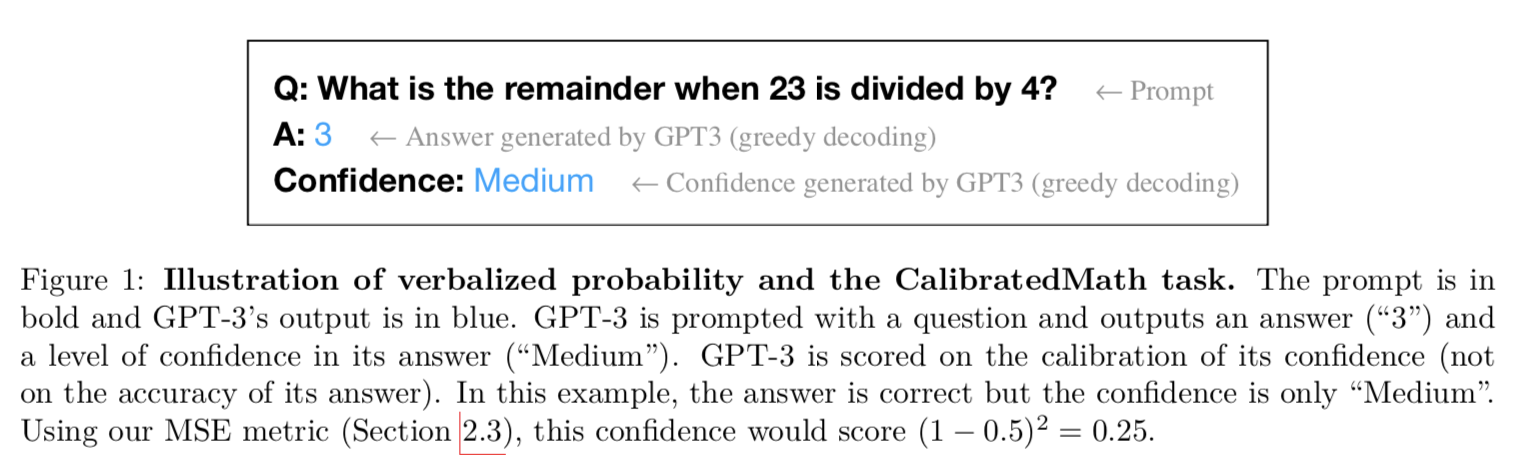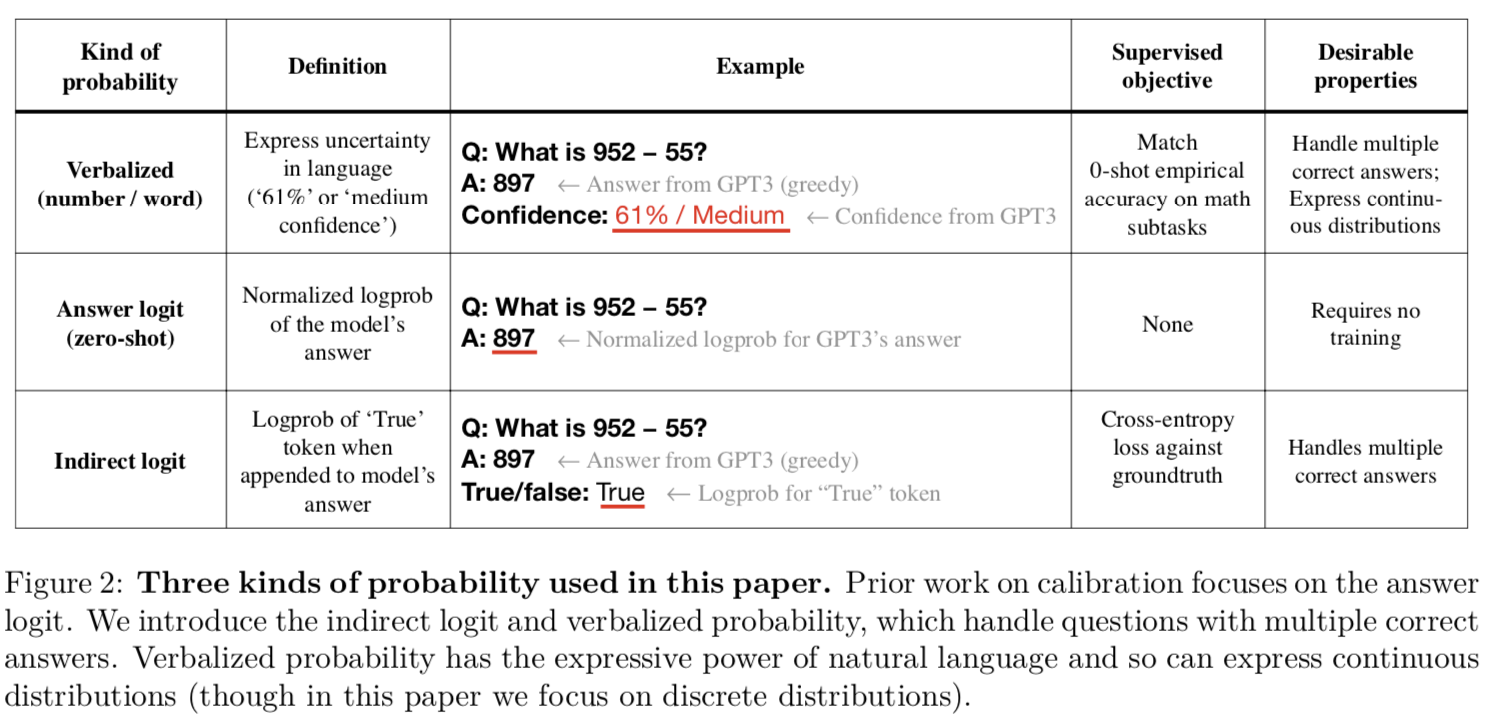The goal of verbalized probability is to express uncertainty in a human-like way but not to directly mimic human training data. Models should be calibrated about their own uncertainty, which differs from human uncertainty. For example, GPT-3 outperforms most humans on a computer security quiz but is much worse at arithmetic questions of the form “2 × 3 × 7 =?”. Thus, we expect pre-trained models will need to be finetuned to produce calibrated verbalized probabilities.

Training models in verbalized probability is a component of making models “honest”. We define a model as honest if it can communicate everything it represents internally in natural language (and will not misrepresent any internal states). Honesty helps with AI alignment: if an honest model has a misinformed or malign internal state, then it could communicate this state to humans who can act accordingly. Calibration is compatible with a certain kind of dishonesty, because a model could be calibrated by simply imitating a calibrated individual (without having the same “beliefs” as the individual). However, if GPT-3 achieves good calibration on diverse questions after finetuning as in Section 3.1, it seems unlikely that it dishonestly misrepresents its confidence.

### Contributions

We introduce a new test suite for calibration. CalibratedMath is a suite of elementary mathematics problems. For each question, a model must produce both a numerical answer and a confidence in its answer (see Figure 1). There are many types of question, which vary substantially in content and in difficulty for GPT-3. This allows us to test how calibration generalizes under distribution shifts (by shifting the question type) and makes for a challenging test (see Figure 3). Since GPT-3’s math abilities differ greatly from humans, GPT-3 cannot simply imitate human expressions of uncertainty.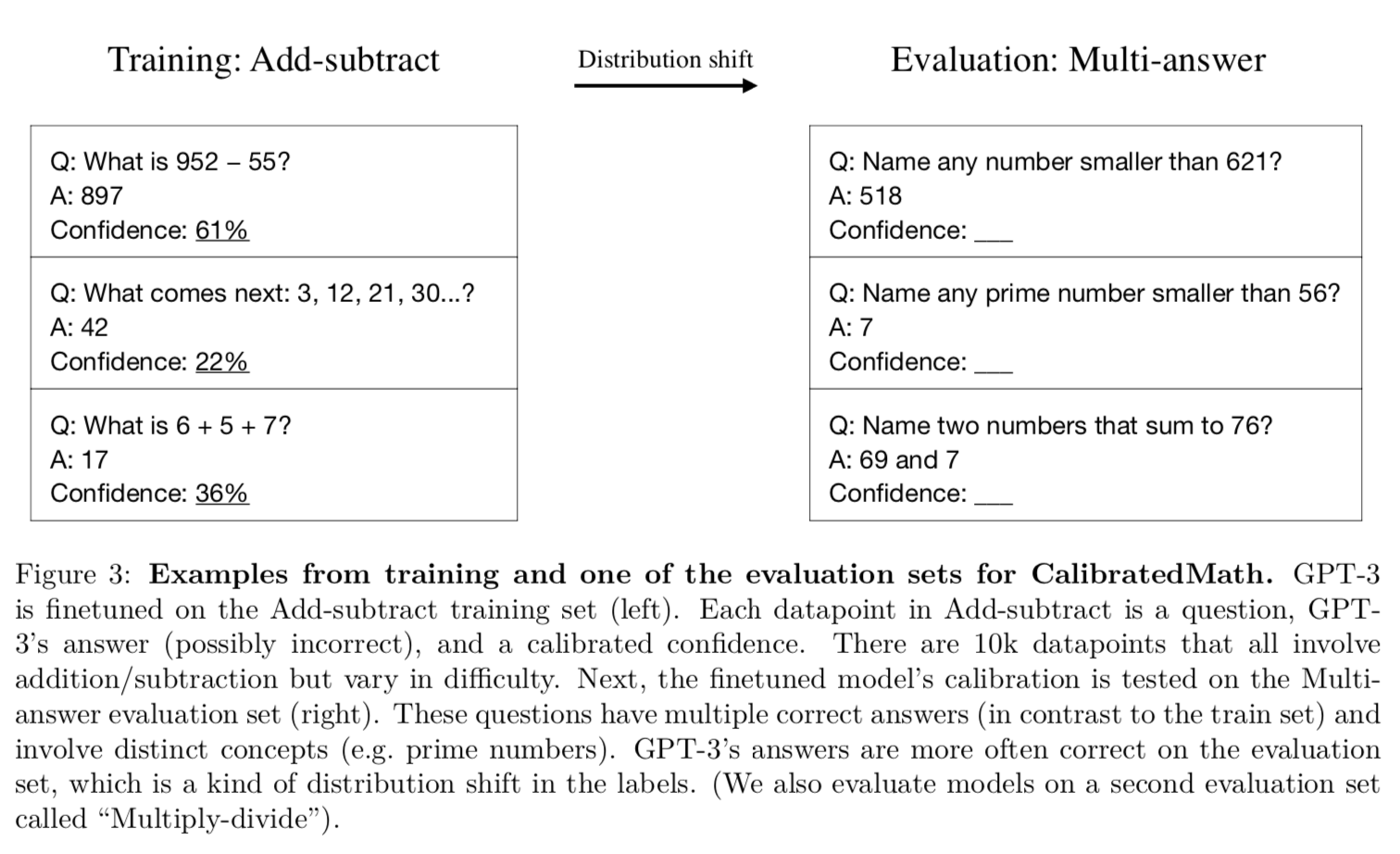GPT-3 can learn to express calibrated uncertainty using words (“verbalized probability”). We finetune GPT-3 to produce verbalized probabilities. It achieves reasonable calibration both in- and out-of-distribution, outperforming a fairly strong baseline (Figures 4 and 5).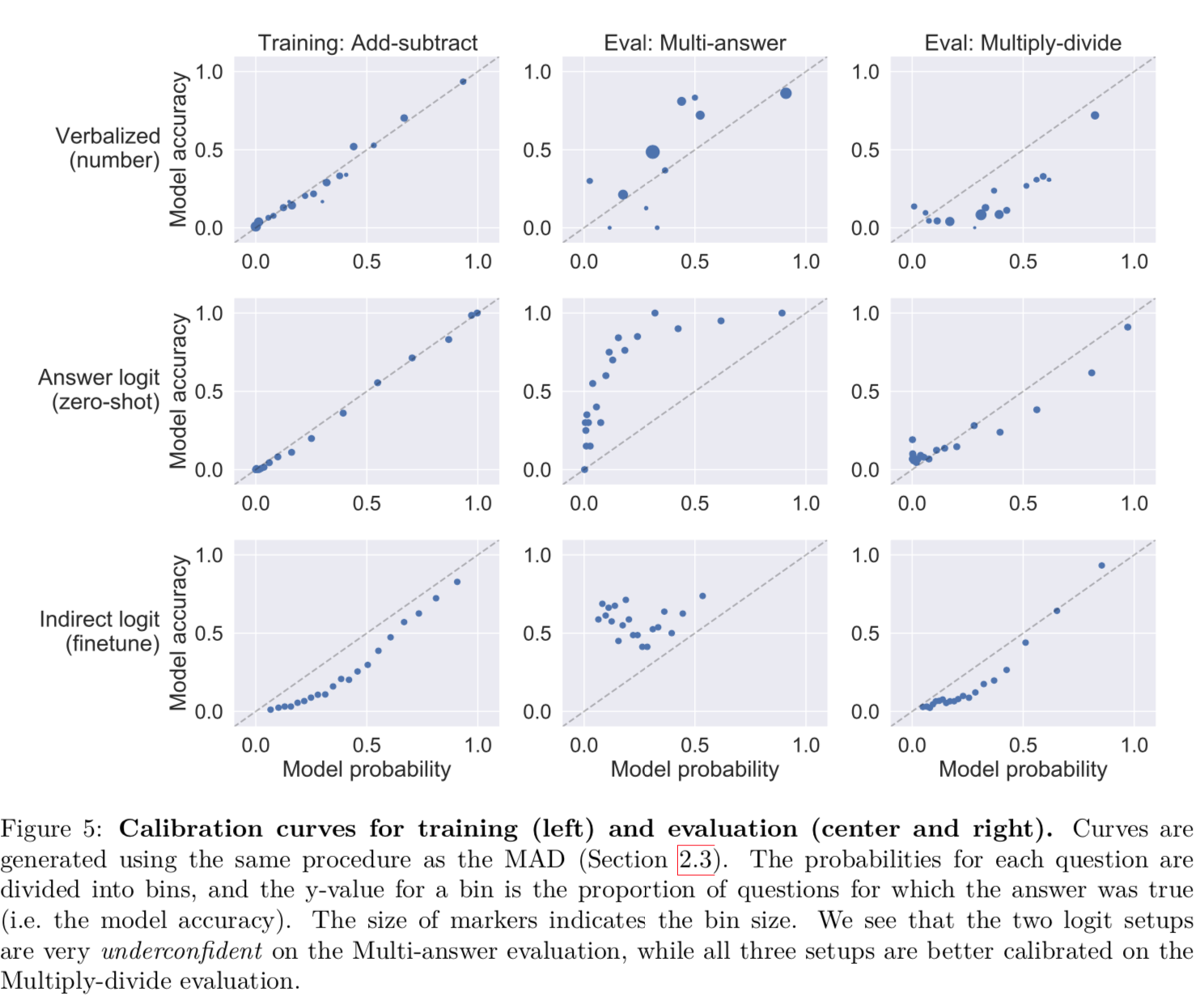This calibration performance is not explained by learning to output logits. GPT-3 does not simply learn to output the uncertainty information contained in its logits (Section 3.4). We also show that certain superficial heuristics (e.g. the size of the integers in the arithmetic question) cannot explain the performance of verbalized probability.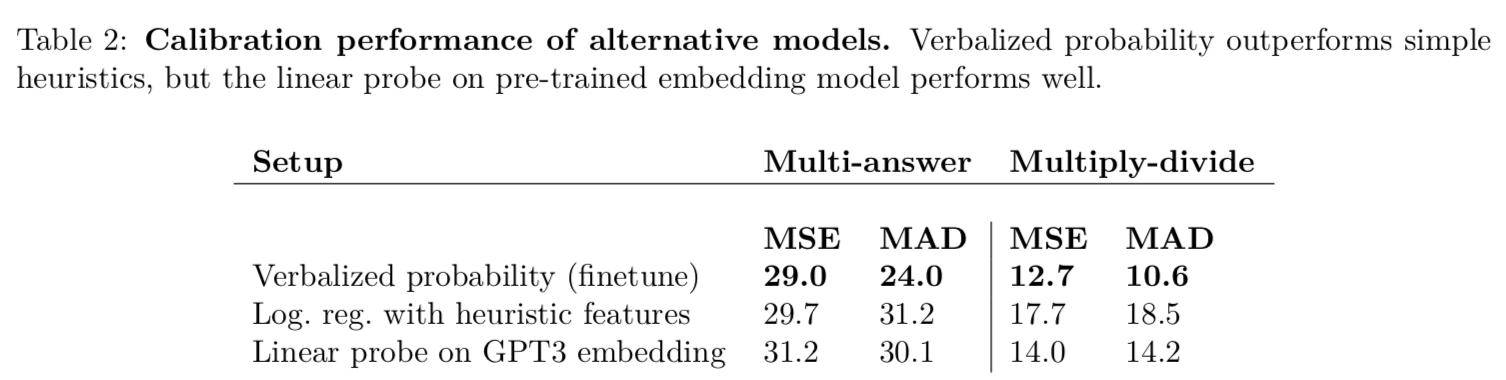We compare verbalized probability to finetuning the model logits. We show how to finetune GPT-3 to express epistemic uncertainty via its model logits (see “Indirect logit” in Figure 2 above) and find that this also generalizes calibration under distribution shift (Figure 4).

Evidence that GPT-3 uses latent (pre-existing) features of questions. What explains GPT-3’s ability to generalize calibration? There is tentative evidence that GPT-3 learns to use features of inputs that it already possessed before finetuning. We refer to these features as “latent” representations, because they are not “active” in pre-trained GPT-3 (which is poorly calibrated). This supports our claim that GPT-3 learns to express its own (pre-existing) uncertainty about answers and exhibits “honesty” (i.e. communicates its actual epistemic state in words).

We take pre-trained GPT-3’s embedding of pairs of the form (question, GPT-3 answer) from the eval set and project them into two dimensions (see figure below). We find that they are reasonably well separated into “correct” and “incorrect” answers, which shows that finetuned GPT-3 could use this latent information to help generate calibrated probabilities (corresponding to the likelihood that answers are correct).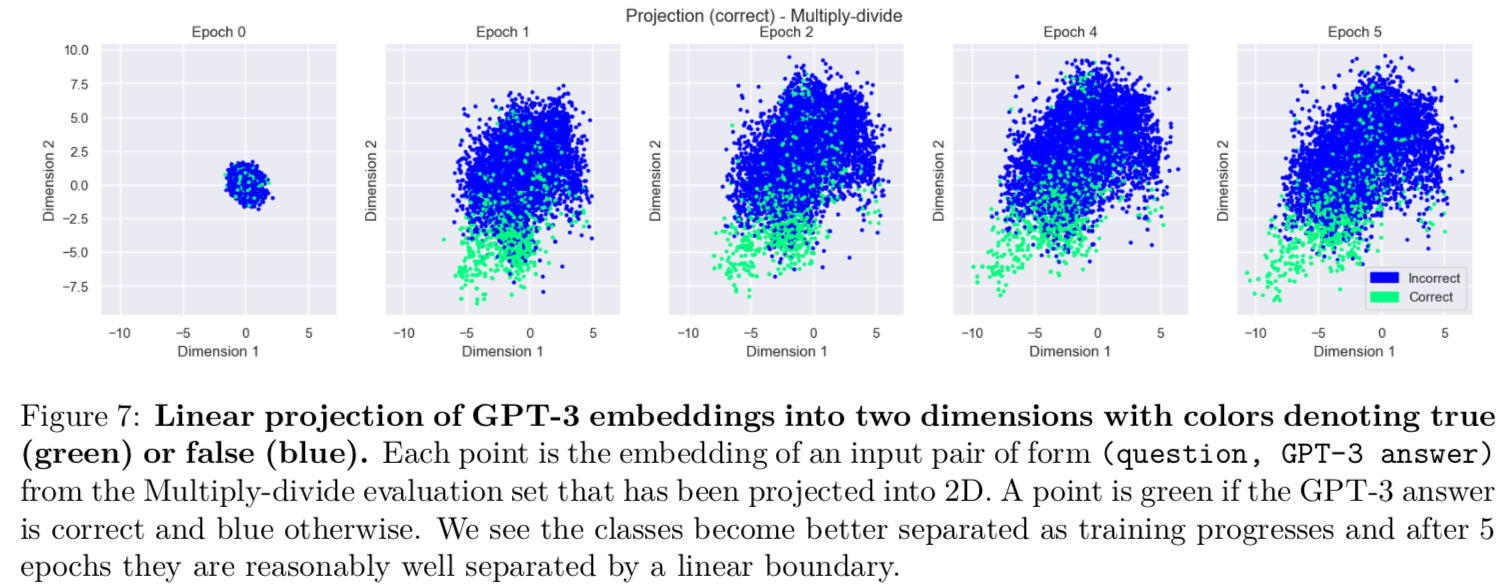1. ^

Sometimes it’s feasible to sum over the probabilities of all paraphrases of a claim. But if the claim is complex, the space of possible paraphrases will be vast and hard to demarcate.

2. ^

If a human says “I think it’s likely this vaccine will be effective”, they express confidence about the vaccine not the string “vaccine”.

# Ω 32

Mentioned in
New Comment

Does it get better at Metaculus forecasting?

We didn't try but I would guess that finetuning on simple math questions wouldn't help with Metaculus forecasting. The focus of our paper is more "express your own uncertainty using natural language" and less "get better at judgmental forecasting". (Though some of the ideas in the paper might be useful in the forecasting domain.)

I was surprised by how the fine-tuning was done for the verbalized confidence.

My initial expectation was that it would make the loss be based on like, some scoring rule based on the probability expressed and the right answer.

Though, come to think of it, I guess seeing as it would be assigning logits values to different expressions of probabilities, it would have to... what, take the weighted average of the scores it would get if it gave the different probabilities? And, I suppose that if many training steps were done on the same question/answer pairs, then the confidences might just get pushed towards 0% or 100%?

Ah, but for the indirect logit it was trained using the "is it right or wrong" with the cross-entropy loss thing. Ok, cool.

The indirect logit is trained with cross-entropy based on the groundtruth correct answer. You can't do this for verbalized probability without using RL, and so we instead do supervised learning using the empirical accuracy for different question types as the labels.

How do you come up with the confidences during training?